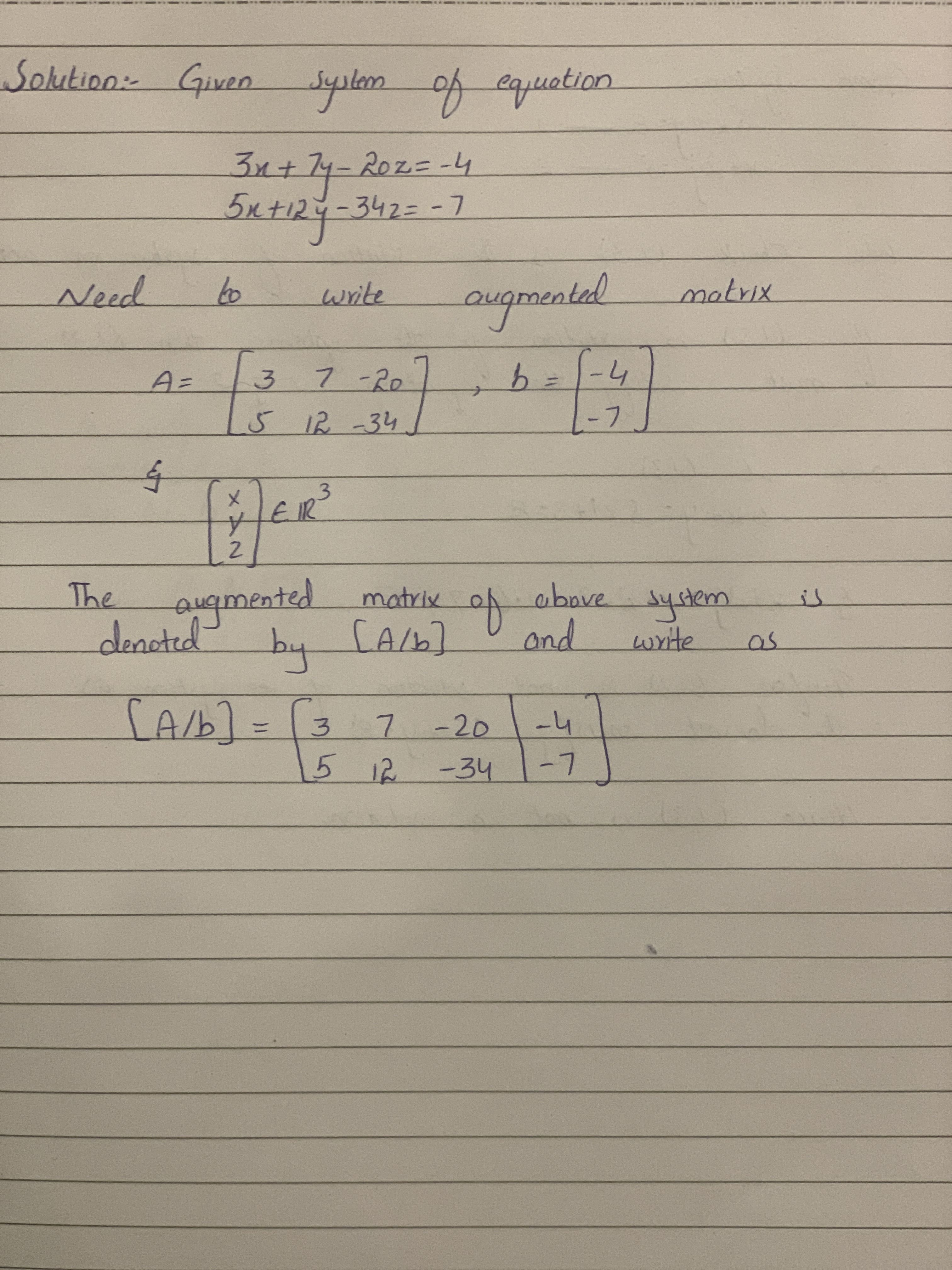# Find the augmented matrix for the following system of linear equations: 3x+7y-20z=-45x+12y-34z=-7Kyran Hudson 2021-03-05 Answered

Find the augmented matrix for the following system of linear equations:
$3x+7y-20z=-4$
$5x+12y-34z=-7$

You can still ask an expert for help

• Questions are typically answered in as fast as 30 minutes

Solve your problem for the price of one coffee

• Math expert for every subject
• Pay only if we can solve itpivonie8..............